# ICSE solutions for Class 10 Physics chapter 7 - Electricity [Latest edition]

#### Chapters## Chapter 7: Electricity

### ICSE solutions for Class 10 Physics Chapter 7 ElectricityShort Answers

State and define Ohm’s law.

Define the unit of resistance (or Define the unit "ohm").

Define ampere and volt with respect to Ohm’s law.

State the limitations of Ohm’s law.

What is non-ohmic resistor?

Define the term electric potential. State its S.I. unit.

Explain, why the potential difference across the terminals of a cell is more when the cell is not in use than it is when the cell is being used.

A cell is used to send current to an external circuit. (a) How does the voltage across its terminals compare with its e.m.f? (b) under what condition is the e.m.f of a cell equal to its terminal
voltage?

State the factors on which the e.m.f of a cell depends.

A cell of e.m.f. E and internal resistance r is used to send current I in an external resistance R. Write expressions for (i) the current drawn from the cell,
(ii) the terminal voltage V of the cell, and
(iii) the voltage drop across the internal resistance. How are E and V related?

Point out two differences between e.m.f. and potential difference as applied to electric circuits.

Explain why potential difference is always less than the e.m.f. of a cell?

Define the term current and state its S.I unit.

What is the voltage of the electricity that is generally supplied to a house?

How is direction of current related to the direction of flow of electrons?

Write an expression for the electrical energy spent in flow of current through an electrical appliance in terms of current, resistance and time.

What do you understand by the resistance of a wire?

State the S.I. unit of electrical resistance and define it.

State the factors on which the resistance of a conductor depends.

How does the resistance of a metallic wire depend on the length of wire?

How does the resistance of a metallic wire depend on the thickness of wire?

How does the resistance of a metallic wire depend on its temperature? Explain with reason.

A given wire is stretched to double its length. How will its resistance change?

A given metallic wire of resistance R is doubled on itself. What will be its new resistance?

How does specific resistance of a semi-conductor change with the increase in temperature?

How does the resistance of an alloy, vary with temperature?

Bends in a rubber pipe reduce the flow of water through it. How would the bands in a wire affect its electric resistance?

State the order of resistivity of (i) a metal, (ii) a semiconductor and (iii) an insulator.

Name the material of wire used for making standard resistances. Give a reason.

What material is used for making wire to prepare heating coils? Give a reason.

Why are thick copper wires used as connecting wires?

State the effect of rise in temperature on the resistance of semi-conductors.

Write an expression for the resistance of a conducting wire in terms of its length and area of cross-section.

How does the resistance of a metallic wire depend on its temperature? Explain with reason.

How does the resistivity of a semiconductor depend on temperature?

How does the resistivity of an alloy such as constantace depends on temperature.

State expression for Cells connected in series.

What is meant by internal resistance of a cell?

Define the term resistivity and state its S.I. unit. Which will have higher resistivity a conductor or an insulator.

The voltage applied to a given rheostat is made four times its initial value. By what factor will be resistance of the rheostat change?

Two copper wires are of the same length, but one is thicker than the other.
(1) Which wire will have more resistance?
(2) Which wire will have more specific resistance?

State expression for Resistance connected in series.

State expression for Resistance connected in parallel.

State expression for Cells connected in parallel.

State the condition when it is advantageous to connect cells in series.

State the condition when it is advantageous to connect cells in parallel.

Name two factors on which the internal resistance of a cell depends and state how does it depend on the factors stated by you.

How should the electric lamps in a building be connected?

What determines the direction of flow of electrons between two charged conductors kept in contact?

What is consumed while using different electrical appliances, for which electricity bills are paid?

Write an expression for calculating electrical power in terms of current and resistance.

Which part of an electrical appliance is earthed?

State the conditions for the flow of charge in a circuit from one point to the other.

An electrical gadget can give an electric shock to its user under certain circumstances. Mention any two of these circumstances.

What is the purpose of fuse in an electrical circuit?

State two characteristics of a fuse wire.

A substance has zero resistance below 1 k. what is such a substance called?

What is an Ohmic resistor?

What is the colour code for the insulation on the earth wire?

State a relation between electrical power, resistance and potential difference in an electrical circuit.

### ICSE solutions for Class 10 Physics Chapter 7 ElectricityLong Answers

What is a resistance? Define it with respect to Ohm’s law,

State and explain the laws of resistance.

### ICSE solutions for Class 10 Physics Chapter 7 ElectricityFigure Based Short Answers

Figure Based Short Answers | Q 1

What are ohmic conductors? Give one exmaple. Draw a graph showing the current-voltage relationship for an ohmic conductor.

Figure Based Short Answers | Q 2

What are non-ohmic conductors? Give one exmaple. Draw a current-voltage graph for a non-ohmic conductor.

Figure Based Short Answers | Q 3

Draw a neat diagram for the verification of Ohm’s law by voltmeter-ammeter method. By another diagram show the relation between p.d. and current.

Figure Based Short Answers | Q 4

A cell of emf. 1.5 V and internal resistance 10 ohms is connected to a resistor of 5 ohms, with an ammeter in series see fig.. What is the reading of the ammeter?

Figure Based Short Answers | Q 5

Draw a graph of Potential difference (V) versus Current (I) for an ohmic resistor. How can you find the resistance of the resistor from this graph?

Figure Based Short Answers | Q 6

The V-I graph for a series combination and for a parallel combination of two resistors is shown in the figure below. Which of the two A or B. represents the parallel combination? Give reasons for your answer.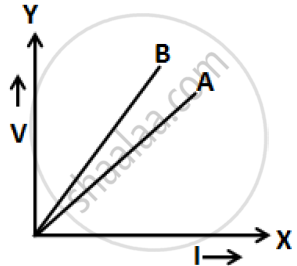Figure Based Short Answers | Q 7

Calculate the effective resistance between the points A and B in the network shown below in Figure 8.45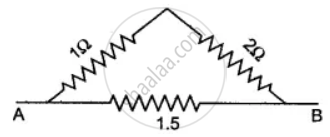Figure Based Short Answers | Q 8

You are provided with three resistors of resistance 1.0 Ω, 2.0 Ω and 3.0 Ω How would you connect them to obtain the total effective resistance 1.5 Ω? Draw diagram of the arrangement and check it by calculations.

Figure Based Short Answers | Q 9

Three resistors each of 2Ω are connected together so that their total resistance is 3Ω. Draw a diagram to show this arrangement and check it by calculation.

Figure Based Short Answers | Q 10

Find the equivalent resistance between points A and B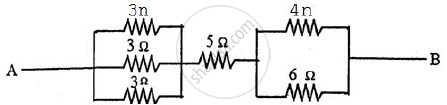Figure Based Short Answers | Q 11

Calculate the equivalent resistance between the points A and B for the following combination of resistors:

Figure Based Short Answers | Q 12

Calculate the equivalent resistance between P and Q from the following diagram:

Figure Based Short Answers | Q 13

Calculate the equivalent resistance between A and B in the adjacent diagram.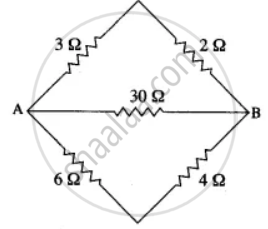Figure Based Short Answers | Q 14

Six resistances are connected together as shown in the figure. Calculate the equivalent resistance between points A and B.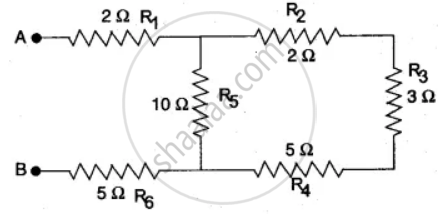Figure Based Short Answers | Q 15

Four resistances of 2.0Ω each are joined end to end, to form a square ABCD. Calculate the equivalent resistance of the combination between any two adjacent comers.

Figure Based Short Answers | Q 16

Three resistors of 6Ω, 3Ω and 2Ω are connected together so that their total resistance is greater than 6Ω but less than 8Ω Draw a diagram to show this arrangement and calculate its total resistance.

Figure Based Short Answers | Q 17

In the circuit shown below, calculate the equivalent resistance between the points (i) A and B, (ii) C and D.

Figure Based Short Answers | Q 18

What is the equivalent resistance between the points X and Y for the given network?

Figure Based Short Answers | Q 19

Six equal resistors of 1 ohm each are connected to form the sides of a hexagon ABCDEF. Calculate the resistance offered by the combination if the current enters at A and leaves it at D.

Figure Based Short Answers | Q 20

Two lamps of resistance 30Ω and 20Ω respectively are connected in series in a 110V circuit. Calculate:
(i) the total resistance in the circuit
(ii) the current in the circuit, and
(iii) the voltage drop across each lamp.

Figure Based Short Answers | Q 21

Calculate the equivalent resistance of the following combination of resistor r1, r2, r3, and r4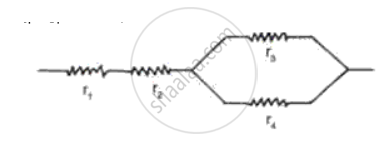Figure Based Short Answers | Q 22

Four cells, each of e.m.f. 1.5 V and internal resistance 2.0 ohms are connected in parallel. The battery of cells is connected to an external resistance of 2.5 ohms. Calculate:

(i) The total resistance of the circuit.
(ii) The current flowing in the external circuit.
(iii) The drop in potential across-the terminals of the cells.

Figure Based Short Answers | Q 23

2Ω resistor A, 1Ω resistor B and 4Ω resistor C are connected in parallel. The combination is connected across a 2V battery of negligible resistance. Draw the diagram of the arrangement and calculate:
(i) The current in each resistor A and C,
(ii) The current through battery.

### ICSE solutions for Class 10 Physics Chapter 7 ElectricityFigure Based Long Answers

Figure Based Long Answers | Q 1

Define the e.m.f. (E) of a cell and the potential difference (V) of a resistor R in terms of the work done in moving a unit charge. State the relation between these two works and the work done in moving a unit charge through a cell connected across the resistor. Take the internal resistance of the cell as ‘r’. Hence obtain an expression for the current i in the circuit.

Figure Based Long Answers | Q 2

A battery of 4 cell, each of e.m.f. 1.5 volt and internal resistance 0.5 Ω is connected to three resistances as shown in the figure. Calculate:
(i) The total resistance of the circuit.
(ii) The current through the cell.
(iii) The current through each resistance.
(iv) The p.d. across each resistance.

Figure Based Long Answers | Q 3

Four cells each of e.m.f. 2V and internal resistance 0.1 Ω are connected in series to an ammeter of negligible resistance, a 1.6 Ω resistor and an unknown resistor R1. The current in the circuit is 2A. Draw a labelled diagram and calculate: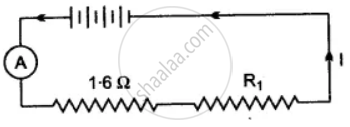(i) Total resistance of the circuit,
(ii) Total e.m.f.
(iii) The value of R1 and
(iv) The p.d. across R1.

Figure Based Long Answers | Q 4

In the figure below, the ammeter A reads 0.3 A. Calculate:
(i) the total resistance of the circuit
(ii) the value of R
(iii) the current flowing through R.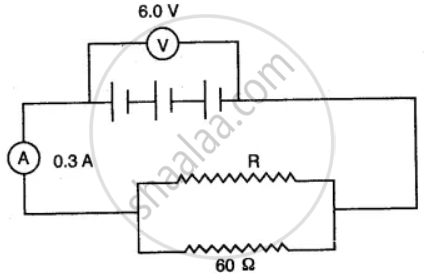Figure Based Long Answers | Q 5

Three resistors are connected to a 12 V battery as shown in the figure given below: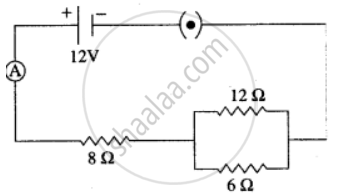(i) What is the current through the 8 ohm resistor?
(ii) What is the potential difference across the parallel combination of 6 ohm and 12 ohm resistor?
(iii) What is the current through the 6 ohm resistor?

Figure Based Long Answers | Q 6

From the following observations taken while determining the resistance of a conductor, draw the current-voltage graph and calculate the resistance of conductor. Is the conductor ohmic?

 Ammeter reading I (in ampere) Voltmeter reading V (in volt) 0.2 0.4 0.3 0.6 0.5 1.0 0.8 1.6 1.5 3.0
Figure Based Long Answers | Q 7

Calculate equivalent resistance in the following cases: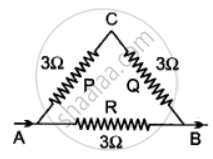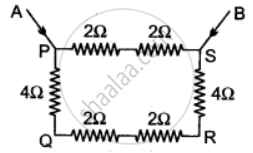Figure Based Long Answers | Q 8

Three resistors are connected to a 6 V battery as shown in the figure given below: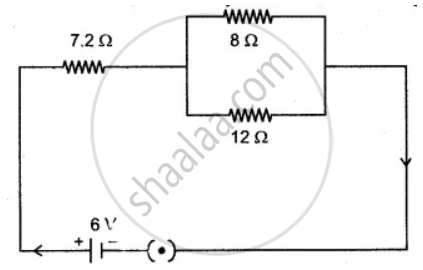Calculate:
(i) the equivalent resistance of the circuit.
(ii) total current in the circuit.
(iii) potential difference across the 7.2 Ω resistor.

Figure Based Long Answers | Q 9

Five resistors of different resistances are connected together as shown in the figure. A 12 V battery is connected to the arrangement.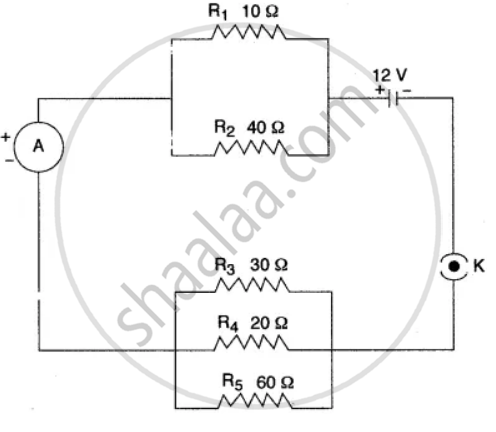Calculate:
(i) the total resistance in the circuit
(ii) the total current flowing in the circuit.

Figure Based Long Answers | Q 10.1

Suppose there are three resistors A, B, and C having resistances r1, r2, and r3 respectively. If R represents their equivalent resistance, establish the following relation R = r1 + r2 + r3 when joined in series.

Figure Based Long Answers | Q 10.2

Suppose there are three resistors A, B, and C having resistances r1, r2, and r3 respectively. If R represents their equivalent resistance, establish the following relation 1/"R" = 1/"r"_1 + 1/"r"_2 + 1/"r"_3, when joined in parallel.

Figure Based Long Answers | Q 11.1

Illustrate-combination of cells e.g., three cells, in series, explaining the combination briefly. Obtain an expression for current ‘i’ in the combination.

Figure Based Long Answers | Q 11.2

Illustrate-combination of cells e.g., three cells, in parallel, explaining the combination briefly. Obtain an expression for current ‘i’ in the combination.

Figure Based Long Answers | Q 12.1

Two resistors of 4Ω and 6Ω are connected in parallel to a cell to draw 0.5 A current from the cell.
Draw a labelled circuit diagram showing the above arrangement.

Figure Based Long Answers | Q 12.2

Two resistors of 4Ω and 6Ω are connected in parallel to a cell to draw 0.5 A current from the cell. Calculate the current in each resistor.

Figure Based Long Answers | Q 13

The figure shows a circuit. When the circuit is switched on, the ammeter reads 0.5 A.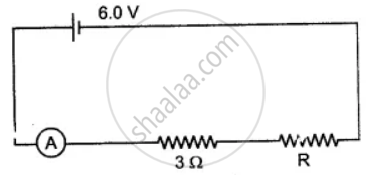(i) Calculate the value of the unknown resistor R.

(ii) Calculate the charge passing through the 3 Ω resistor in 120 s.

(iii) Calculate the power dissipated in the 3 Ω resistor.

Figure Based Long Answers | Q 14

In the figure given below, A, B and C are three ammeters. The ammeter B reads 0.5A. (All the ammeters have negligible resistance.)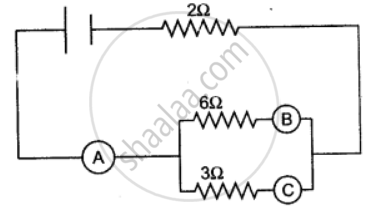Calculate:
(i) the readings in the ammeters A and C.
(ii) the total resistance of the circuit.

Short Numericals

### ICSE solutions for Class 10 Physics Chapter 7 ElectricityShort Numericals

Short Numericals | Q 1

A cell supplies a current of 0.6 A through a 2Ω coil and a current of 0.3 A through on 8Ω coil. Calculate the e.m.f and internal resistance of the cell.

Short Numericals | Q 2

A cell of e.m.f 2.0 V and internal resistance 1Ω is connected to the resistors of 3Ω and 6Ω in series. Calculate:
(i) the current drawn from the cell,
(ii) the p.d. across each resistor,
(iii) the terminal voltage of the cell and
(iv) the voltage drop.

Short Numericals | Q 3

A current of 0.2 A flows through a conducting wire for 5 minutes. How much charge will flow?

Short Numericals | Q 4

A current of 100 mA. flows through a wire. The charge on an electron is 1.6 × 10-19 C. Find the number of electrons passing per second through the cross-section of the conductor.

Short Numericals | Q 5

An electric bulb draws 0.5 A current at 3.0 V. Calculate the resistance of the filament of the bulb.

Short Numericals | Q 6

Two bulbs are marked 100 W, 220 V and 60 W, 110 V. Calculate the ratio of their resistances.

Short Numericals | Q 7

An ammeter placed in series with an electric radiator reads 0.5 amps and a voltmeter placed across it reads 230 volts. What is the resistance of the radiator?

Short Numericals | Q 8

What should be the length of a nickel wire of area of cross-section 3 mm2 used for making a rheostat of 750 ohm? (ρ of nickle = 0.069 Ohm mm2/m)

Short Numericals | Q 9

Calculate the value of the resistance which must be connected to a 15 ohm resistance to provide on effective resistance of 6 ohm.

Short Numericals | Q 10

A metal wire of resistance 6 Ω is stretched so that its length is increased to twice its original length. Calculate its new resistance.

Short Numericals | Q 11

Calculate the quantity of heat that will be produced in a coil of resistance 75 Ω if a current of 2A is passed through it for 2 minutes.

Short Numericals | Q 12

Two wires of same material and same lengths have radii in the ratio of 2 : 3, Compare their resistances.

Short Numericals | Q 13

Two resistors having 2Ω and 3Ω resistance are connected—(i) in series, and (ii) in parallel. Find the equivalent resistance in each case.

Short Numericals | Q 14

A wire of uniform thickness with a resistance of 27Ω is cut into three equal pieces and they are joined in parallel. Find the equivalent resistance of the parallel combination.

Short Numericals | Q 15

The resistance of two resistors joined in series is 8Ω and in parallel is 1.5Ω. Find the value of the two resistances.

Short Numericals | Q 16

An electrical appliance is rated at 1000 KVA, 220V. If the appliance is operated for 2 hours, calculate the energy consumed by the appliance in: (i) kWh (ii) joule.

Short Numericals | Q 17.1

An electric bulb is marked '100 W, 250 V'. What information does this convey?

Short Numericals | Q 17.2

An electric bulb is marked 100 W, 250 V. How much current will the bulb draw if connected to a 250 V supply?

Long Numericals

### ICSE solutions for Class 10 Physics Chapter 7 ElectricityLong Numericals

Long Numericals | Q 1

A cell of e.m.f. 1.5 V and internal resistance 1.0 W is connected to two resistors of 4.0 W and 20.0 in series as shown in the figure: Calculate the:

(i) Current in the circuit.
(ii) Potential difference across the 4.0 ohm resistor.
(iii) Voltage drop when the current is flowing.
(iv) Potential difference across the cell.

Long Numericals | Q 2.1

A 10 m long wire of a particular material is of reistance 5Ω What will be the resistance of 5m long wire of same material and the same thickness.

Long Numericals | Q 2.2

A 10 m long wire of a particular material is of resistance 5Ω What will be the resistance of 10m long wire of the same material but the double radius.

Long Numericals | Q 2.3

A 10 m long wire of a particular material is of resistance 5Ω What will be the resistance if the wire is doubled itself.

Long Numericals | Q 2.4

A 10 m long wire of a particular material is of resistance 5Ω What will be the resistance if the wire is stretched to double its length?

Long Numericals | Q 3

Three resistances, of which two are equal when connected in series have an effective resistance of 30 ohms. When the three resistances are connected in parallel, the effective resistances is 3 ohms. Find the values of the individual resistances.

Long Numericals | Q 4

When a resistance of 3Ω is connected across a cell, the current flowing is 0.5 A. On changing the resistance to 7Ω, the current becomes 0.25A. Calculate the e.m.f. and the internal resistance of the cell.

## Chapter 7: Electricity## ICSE solutions for Class 10 Physics chapter 7 - Electricity

ICSE solutions for Class 10 Physics chapter 7 (Electricity) include all questions with solution and detail explanation. This will clear students doubts about any question and improve application skills while preparing for board exams. The detailed, step-by-step solutions will help you understand the concepts better and clear your confusions, if any. Shaalaa.com has the CISCE Class 10 Physics solutions in a manner that help students grasp basic concepts better and faster.

Further, we at Shaalaa.com provide such solutions so that students can prepare for written exams. ICSE textbook solutions can be a core help for self-study and acts as a perfect self-help guidance for students.

Concepts covered in Class 10 Physics chapter 7 Electricity are Ohm's Law, Resistance (R), Electrical Resistivity and Electrical Conductivity, Ohmic and Non-ohmic Resistors, Choice of Material of a Wire, Superconductors, Electro-motive Force (E.M.F.) of a Cell, Terminal Voltage of a Cell, Internal Resistance of a Cell, Experimental Verification of Ohm’s Law, Electric Current, Resistors in Series, System of Resistors, Resistances in Parallel, Series Connection of Parallel Resistors, Parallel Connection of Series Resistors, Electric Circuit, Electric Charge, Electric Potential (Electrostatic Potential) and Potential Difference.

Using ICSE Class 10 solutions Electricity exercise by students are an easy way to prepare for the exams, as they involve solutions arranged chapter-wise also page wise. The questions involved in ICSE Solutions are important questions that can be asked in the final exam. Maximum students of CISCE Class 10 prefer ICSE Textbook Solutions to score more in exam.

Get the free view of chapter 7 Electricity Class 10 extra questions for Class 10 Physics and can use Shaalaa.com to keep it handy for your exam preparation